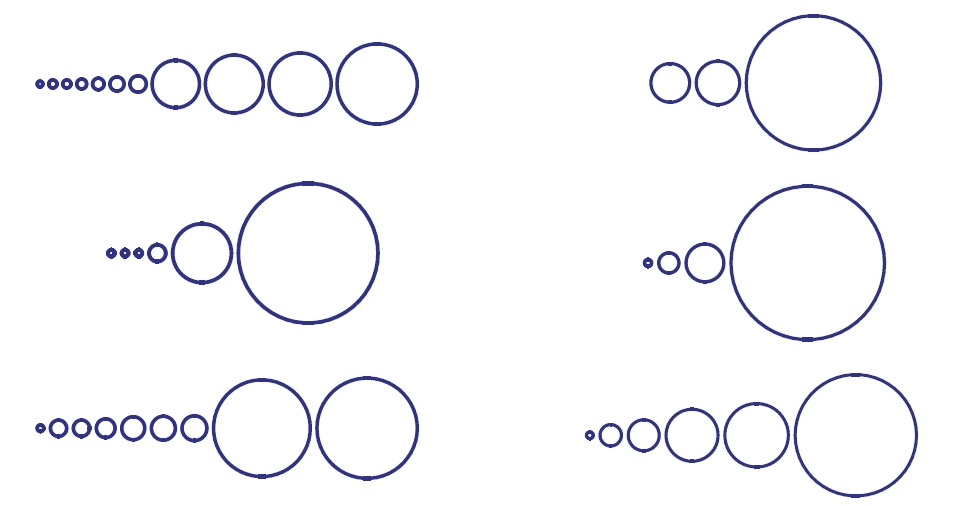Random Permutations

## Random Permutations

#### John BaezSix random permutations of a 500-element set, where
circle areas are drawn in proportion to cycle lengths.
From Analytic Combinatorics by Flajolet and Sedgewick.

Say you randomly choose one of the $$n!$$ permutations of a huge $$n$$-element set. What's it like, typically? This is actually many questions in one. Answering these questions uses a fascinating mix of elementary techniques, generating functions, and complex analysis. And the answers are often beautifully simple!

• Part 0 — What's the average length of the longest cycle in a random permutation of an $$n$$-element set?
• Part 1 — What is the probability that a randomly chosen permutation of an $$n$$-element set has exactly $$k$$ fixed points?

• Part 2 — What is the probability that the shortest cycle in a randomly chosen permutation of an $$n$$-element set has length greater than $$k$$?

• Part 3 — A large collection of questions about random permutations, with answers.
• Part 4 — What is the probability that a randomly chosen permutation of an $$n$$-element set has a cycle of length greater than $$n/2$$?

• Part 5 — What is the average length of a cycle in a randomly chosen permutation of an $$n$$-element set?
• Part 6 — What expected number of cycles of length $$k$$ in a randomly chosen permutation of an $$n$$-element set?
• Part 7 — How is the distribution of the number of cycles of length $$k$$ in a random permutation related to a Poisson distribution?
• Part 8 — What's the $$n$$th moment of a Poisson distribution?
My own arguments emphasize the use of combinatorial species and their generating functions, so it will help if you know a bit about those. These books are helpful: